Engineering Mechanics - PKRB: Work and Energy

Why Engineering Mechanics PKRB: Work and Energy?

In this section you can learn and practice Engineering Mechanics Questions based on "PKRB: Work and Energy" and improve your skills in order to face the interview, competitive examination and various entrance test (CAT, GATE, GRE, MAT, Bank Exam, Railway Exam etc.) with full confidence.

Where can I get Engineering Mechanics PKRB: Work and Energy questions and answers with explanation?

IndiaBIX provides you lots of fully solved Engineering Mechanics (PKRB: Work and Energy) questions and answers with Explanation. Solved examples with detailed answer description, explanation are given and it would be easy to understand. All students, freshers can download Engineering Mechanics PKRB: Work and Energy quiz questions with answers as PDF files and eBooks.

Where can I get Engineering Mechanics PKRB: Work and Energy Interview Questions and Answers (objective type, multiple choice)?

Here you can find objective type Engineering Mechanics PKRB: Work and Energy questions and answers for interview and entrance examination. Multiple choice and true or false type questions are also provided.

How to solve Engineering Mechanics PKRB: Work and Energy problems?

You can easily solve all kind of Engineering Mechanics questions based on PKRB: Work and Energy by practicing the objective type exercises given below, also get shortcut methods to solve Engineering Mechanics PKRB: Work and Energy problems.

Exercise :: PKRB: Work and Energy - General Questions

• PKRB: Work and Energy - General Questions
1.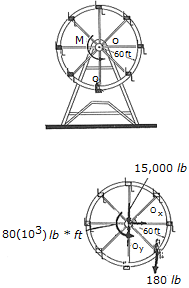A man having a weight of 180 lb sits in a chair of the Ferris wheel, which has a weight of 15,000 lb and a radius of gyration of ko = 37 ft. If a torque of M = 80(103) lb • ft is applied about O, determine the angular velocity of the wheel after it has rotated 180°. Neglect the weight of the chairs and note that the man remains in an upright position as the wheel rotates. The wheel starts from rest in the position shown.

 A.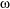= 0.888 rad/s B.= 0.836 rad/s C.= 0.874 rad/s D.= 0.849 rad/s

Explanation:

No answer description available for this question. Let us discuss.

2.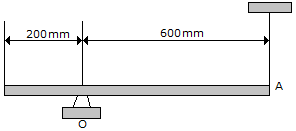The uniform slender rod has a mass of 5 kg. Determine the magnitude of the reaction at the pin O when the cord at A is cut and= 90°

 A. O = 42.0 N B. O = 91.1 N C. O = 122.6 N D. O = 67.4 N

Explanation:

No answer description available for this question. Let us discuss.

3.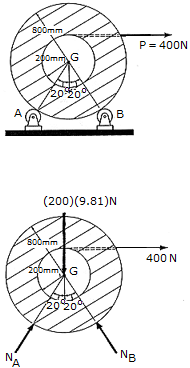The spool of cable, originally at rest, has a mass of 200 kg and a radius of gyration of kG = 325 mm. If the spool rests on two small rollers A and B and a constant horizontal force of P = 400 N is applied to the end of the cable, compute the angular velocity of the spool when 8 m of cable has been unraveled. Neglect friction and the mass of the rollers and unraveled cable.

 A.= 10.00 rad/s B.= 12.31 rad/s C.= 17.41 rad/s D.= 40.0 rad/s

Explanation:

No answer description available for this question. Let us discuss.

4.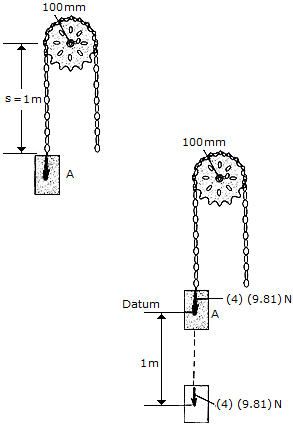A chain that has a negligible mass is draped over a sprocket which has a mass of 2 kg and a radius of gyration of kO = 50 mm. If the 4-kg block A is released from rest in the position shown, s = 1 m, determine the angular velocity which the chain imparts th the sprocket when s = 2 m.

 A.= 44.3 rad/s B.= 39.6 rad/s C.= 41.8 rad/s D.= 59.1 rad/s

Explanation:

No answer description available for this question. Let us discuss.

5.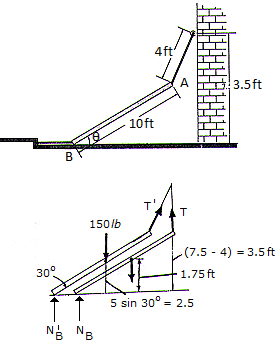The beam having a weight of 150 lb is supported by two cables. If the cable at end B is cut so that the beam is released from rest when= 30°, determine the speed at which end A strikes the wall. Neglect friction at B. Consider the beam to be a thin rod.

 A. vA = 5.87 ft/s B. vA = 7.43 ft/s C. vA = 10.18 ft/s D. vA = 6.95 ft/s